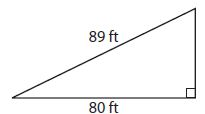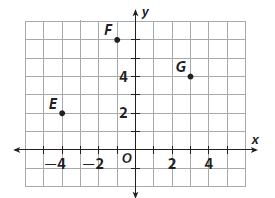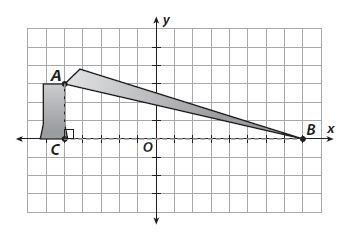Homework Explained - Math Practice 101Dear guest, you are not a registered member. As a guest, you only have read-only access to our books, tests and other practice materials.

As a registered member you can:

Registration is free and doesn't require any type of payment information. Click here to Register.
Go to page:
Chapter 12:The Pythagorean Theorem; Mixed Review

### Selected Response

• Question 1

What is the missing length of the side?• A. 9 ft
• B. 30 ft
• C. 39 ft
• D. 120 ft
• Question 2

Which relation does not represent a function?

• A. (0, 8), (3, 8), (1, 6)
• B. (4, 2), (6, 1), (8, 9)
• C. (1, 20), (2, 23), (9, 26)
• D. (0, 3), (2, 3), (2, 0)
• Question 3

Two sides of a right triangle have lengths of 72 cm and 97 cm. The third side is not the hypotenuse. How long is the third side?

• A. 25 cm
• B. 45 cm
• C. 65 cm
• D. 121 cm
• Question 4

To the nearest tenth, what is the distance between point F and point G?• A. 4.5 units
• B. 5.0 units
• C. 7.3 units
• D. 20 units
• Question 5

A flagpole is 53 feet tall. A rope is tied to the top of the flagpole and secured to the ground 28 feet from the base of the flagpole. What is the length of the rope?

• A. 25 feet
• B. 45 feet
• C. 53 feet
• D. 60 feet
• Question 6

Which set of lengths are not the side lengths of a right triangle?

• A. 36, 77, 85
• B. 20, 99, 101
• C. 27, 120, 123
• D. 24, 33, 42
• Question 7

A triangle has one right angle. What could the measures of the other two angles be?

• A. $$25^0 \text{ and }65^0$$
• B. $$30^0\text{ and }15^0$$
• C $$55^0 \text{ and } 125^0$$
• D $$90^0\text{ and }100^0$$

• Question 8

A fallen tree is shown on the coordinate grid below. Each unit represents 1 meter.a. What is the distance from A to B?

•  meters
• Question 8

b. What was the height of the tree before it fell?

•  meters

Yes, email page to my online tutor. (if you didn't add a tutor yet, you can add one here)